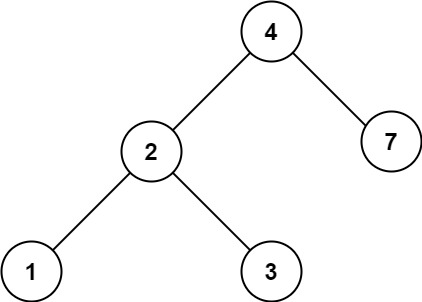## 700. Search in a Binary Search Tree

You are given the `root` of a binary search tree (BST) and an integer `val`.

Find the node in the BST that the node's value equals `val` and return the subtree rooted with that node. If such a node does not exist, return `null`.

Example 1:```Input: root = [4,2,7,1,3], val = 2
Output: [2,1,3]
```

Example 2:```Input: root = [4,2,7,1,3], val = 5
Output: []
```

Constraints:

• The number of nodes in the tree is in the range `[1, 5000]`.
• `1 <= Node.val <= 107`
• `root` is a binary search tree.
• `1 <= val <= 107`

## Rust Solution

``````struct Solution;
use rustgym_util::*;

trait Search {
fn find(&self, val: i32) -> TreeLink;
}

fn find(&self, val: i32) -> TreeLink {
if let Some(node) = self {
let temp = node.clone();
let node = node.borrow();
if val == node.val {
Some(temp)
} else {
if val < node.val {
Self::find(&node.left, val)
} else {
Self::find(&node.right, val)
}
}
} else {
None
}
}
}

impl Solution {